# WEST Math: Matrix Operations & Determinants Chapter Exam

Exam Instructions:

Choose your answers to the questions and click 'Next' to see the next set of questions. You can skip questions if you would like and come back to them later with the yellow "Go To First Skipped Question" button. When you have completed the practice exam, a green submit button will appear. Click it to see your results. Good luck!

Answered 0 of 30

### Page 1

#### Question 2 2. If you switch rows two and three, what will be the new second row?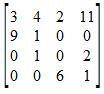#### Question 3 3. Which row would you multiply by -2 to help you find the inverse matrix?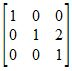#### Question 5 5. Find the determinant of the following matrix.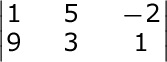### Page 2

#### Question 6 6. What is the number in the second row and third column in the answer matrix?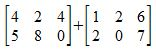#### Question 8 8. Which number needs to be changed to find the inverse of this matrix?### Page 3

#### Question 15 15. Which of the following is the inverse of this matrix?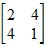### Page 4

#### Question 16 16. Multiply the first row by -3 and add it to the second row.#### Question 18 18. What size is the following matrix?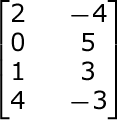#### Question 19 19. Multiply the fourth row by 3.#### Question 20 20. Which of the following is the new row that results when you add rows 1 and 3?### Page 5

#### Question 21 21. Find the determinant of the following matrix.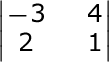### Page 6

#### Question 26 26. Which system of equations is represented by the following matrix? (Think of the vertical line as the equal sign.)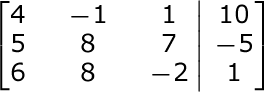#### Question 27 27. What is the number in the first row and first column in the answer matrix?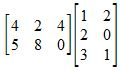#### Question 28 28. If you switch the first row with the fourth row, what will the new first row be?#### Question 30 30. If the inverse of matrix A is this, what is the solution when the constants are 10 and 13?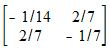#### WEST Math: Matrix Operations & Determinants Chapter Exam Instructions

Choose your answers to the questions and click 'Next' to see the next set of questions. You can skip questions if you would like and come back to them later with the yellow "Go To First Skipped Question" button. When you have completed the practice exam, a green submit button will appear. Click it to see your results. Good luck!

Support